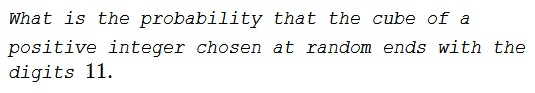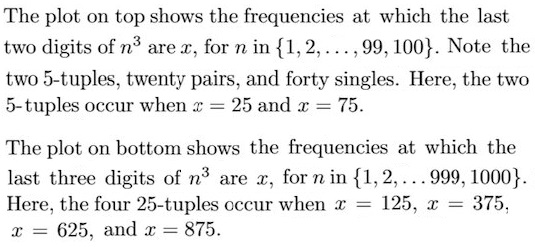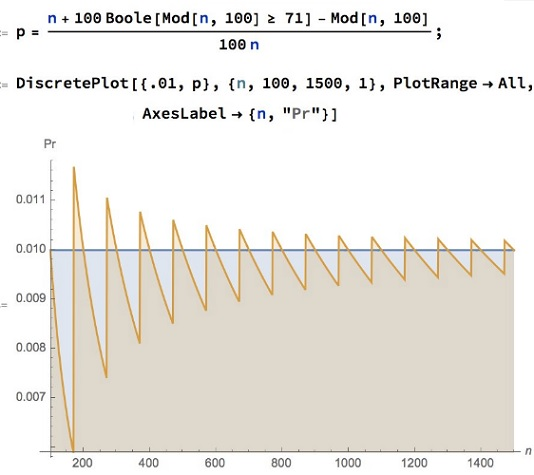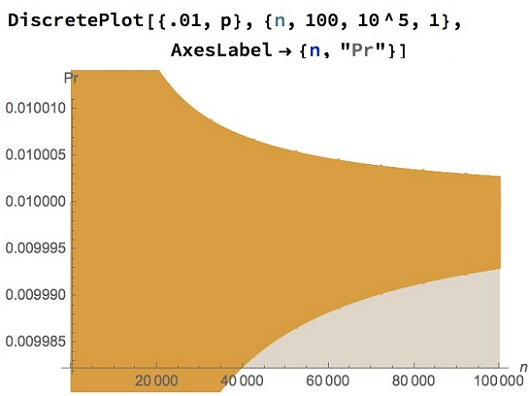Probability of a Cube Ending with 11

ProblemSolution 1

If $a^3$ ends in the digits $11,$ then $a^3\equiv 11\,(\text{mod }100).$ This is equivalent to the following two conditions:

1. $a^3\equiv 11\equiv 3\,(\text{mod }4)$ and
2. $a^3\equiv 11\,(\text{mod }25).$

Easily, $3$ is the only residue modulo $4$ that satisfies $a^3\equiv 3\,(\text{mod }4).$

Next, $a^3\equiv 11\,(\text{mod }25)$ implies $a^3\equiv 1\,(\text{mod }5).$ Checking the residues, $1$ is the only one that satisfies this condition. From here, suffice it to check $1,$ $6,$ $11,$ $16,$ $21$ modulo $25:$

$1^3=1\equiv 1\,(\text{mod }25)\\ 6^3=200+16\equiv 16\,(\text{mod }25)\\ 11^3=121\cdot 11\equiv 21\cdot 11=231\equiv 6\,(\text{mod }25)\\ 16^3=256\cdot 16\equiv 6\cdot 16\equiv 21\,(\text{mod }25)\\ 21^3=441\cdot 21\equiv 16\cdot 21=210+126\equiv 10+1=11\,(\text{mod }25)$

Thus we are looking for an integer $a$ such that $a\equiv 3\,(\text{mod }4)$ and $a\equiv 21\,(\text{mod }25).$ By the Chinese Remainder Theorem we find that the only number below $75$ satisfying the two equations is $71.$ The only candidate for $a\equiv 21\,(\text{mod }25)$ below $100$ is $96$ that fails $a\equiv 3\,(\text{mod }4).$ It follows that $71$ is the only solution to $a^3\equiv 11\,(\text{mod }100).$ We conclude that the probability of randomly hitting on a solution to $a^3\equiv 11\,(\text{mod }100)$ is $\displaystyle \frac{1}{100}.$

Solution 2

The number ends with $1.$ The form is $(10x + 1)^3$ or $1000x^3 + 300x^2 + 30x + 1.$ $30x$ fixes the $10th$ place and $x=7$ is unique integer solution. So, $71^3\equiv 11\,(\text{mod }100)$ and is the only number below $100$ with this property. The probability of hitting it equals $\displaystyle \frac{1}{100}.$

Solution 3

Since $x^3 \equiv 11$ (mod $100$) implies $x^3 \equiv 1$ (mod $10$), we may begin by narrowing our attention to $x \in \{1, 11, 21, \dots , 91 \}$.

Now make the substitution $a = x-1$. Then we must solve

$(a+1)^3 \equiv 11\quad (\text{mod }100)$

for $a\in\{10,20, \dots, 90 \}$. Note that, for any such $a$, this congruence becomes

\begin{align} &a^3 + 3a^2 + 3a + 1 \equiv 11 \quad (\text{mod } 100)\;\Longrightarrow\\ &3a^2 + 3a \equiv 10 \quad (\text{mod } 100). \end{align}

Now make the substitution $b= a/10$. Then, for $b \in \{1,2, \dots, 9\}$, we must solve

\begin{align} & 3(10b)^2 + 3(10b) \equiv 10 \quad (\text{mod } 100)\;\Longrightarrow\\ & 30 b \equiv 10 \quad (\text{mod } 100) . \end{align}

By inspection, this yields $b = 7$, which gives $a = 70$, which gives $x = 71$.

Solution 4

\displaystyle \begin{align} &x^3\equiv 11~(mod~4) \;\Rightarrow\; x^3\equiv 3~(mod~4)\;\Rightarrow\; x\equiv 3~(mod~4) \\ &x^3\equiv 11~(mod~5) \;\Rightarrow\; x^3\equiv 1~(mod~5)\;\Rightarrow\; x\equiv 1~(mod~5). \end{align}

The only numbers less than equal to $100$ that satisfy these two conditions belong to the set $P=\{11,31,51,71,91\}$. These numbers are respectively $11,6,1,21,16~(mod~25)$. The only case that satisfies $x^3\equiv 11~(mod~25)$ is $x\equiv 21~(mod~25)$. Thus, $x\equiv 71~(mod~100)$.

The required probability is 1 part in 100.

Acknowledgment

This is problem 6 from the 1982 Indiana College Mathematics Competition, see A Friendly Mathematics Competition by Rick Gillman (ed.) Solution 1 is from the book; Solution 2 is by Sriram Natarajan; Solution 3 is by Jim Henegan, Solution 4 is by Amit Itagi. Illustration is by N. N. Taleb.

Remark

Sam Walters has remarked that not every residue modulo $100$ may belong to an integer cube. Being able to select only among those that do will wind up the probability of hitting on the solution. Indeed, there are only $62$ distinct residues modulo $100.$

There are $40$ residues that are attained by unique numbers below $100.$ These are obtained by numbers ending with $1,3,7,9.$ There are $20$ residues with the second digit even, non-zero. These are attained by pairs of numbers with the difference of $50$ between them. E.g. $32^3=327\mathbf{68}$ and $82^3=5513\mathbf{68}.$ Finally, the ending of $25$ is attained by $5,25,45,65,85$ and the ending of $75$ by $15,35,55,75,95.$

Jim Henegan put together an illuminating graph:Alexandre Borovik adds the following remark:

Luckily, in this problem we have a natural probability measure. Interestingly, for the 7th, 11th, 13th powers answer is the same.

Illustration

For the probability $p$ to be $\displaystyle \frac{1}{100},$ one has to further assume that the support $[0,n]$ is either very large or $n$ is a multiple of $100.$ Now, if the sample space is $[0,150],$ the probability is $\displaystyle \frac{1}{150},$ not $\displaystyle \frac{1}{100}.$ But if the sample space is $[0,171],$ $\displaystyle p=\frac{2}{171}.$ Hence, in general,

$\displaystyle p=\fracn+100\cdot\mathbb{1}_{[n\,\text{mod }100]\,\gt 71}-(n\,\text{mod }100)}100n}$

$n\ge 100.$

Let's visualize: